# Multiple Aggregation Prediction Algorithm (MAPA)

By | April 19, 2014

MAPA code for R is available on CRAN.
An online interactive demo of MAPA can be found here.

Here is a quick demonstration what you can do with the code. The easiest way to produce a forecast with MAPA is to use the mapasimple function.

```> mapasimple(admissions)
t+1      t+2      t+3      t+4      t+5      t+6      t+7      t+8      t+9     t+10     t+11     t+12
457438.0 446869.3 450146.7 462231.5 457512.8 467895.1 457606.0 441295.7 471611.2 454282.0 458308.0 453472.5```

This also gives you a simple plot of the series and the forecast: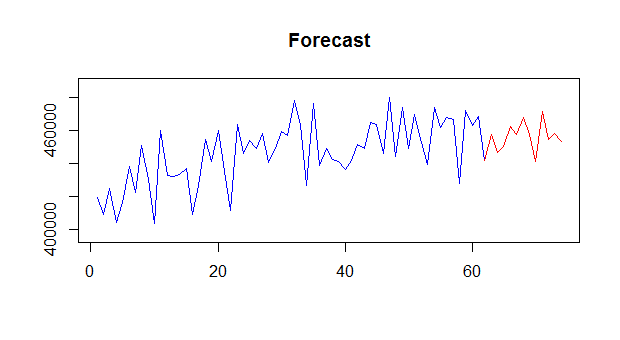I can ask for a detailed view of the predicted states at each temporal aggregation level:

`> mapasimple(admissions,outplot=2,paral=2)`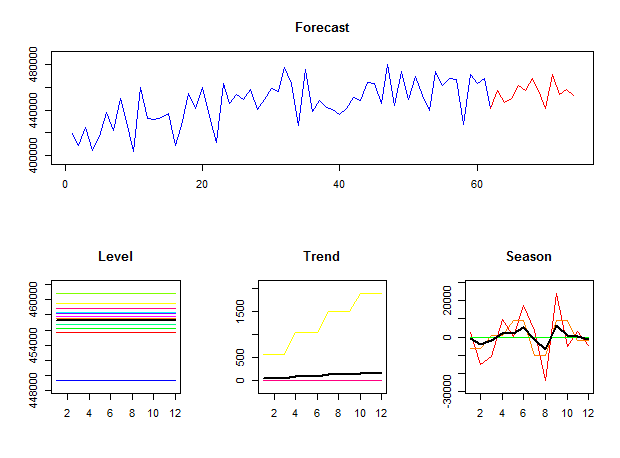In this example I also used paral=2. This creates a parallel cluster, executes mapasimple and then closes the cluster. If you already have a parallel cluster running you can use paral=1.
Using functions mapaest and mapafor I can have a more detailed control of the estimation and the forecasts across the different levels of temporal aggregation.

```> mapafit <- mapaest(admissions,paral=2)

The first function estimates the model fit at each temporal aggregation level and also provides a visualisation of the identified ETS components.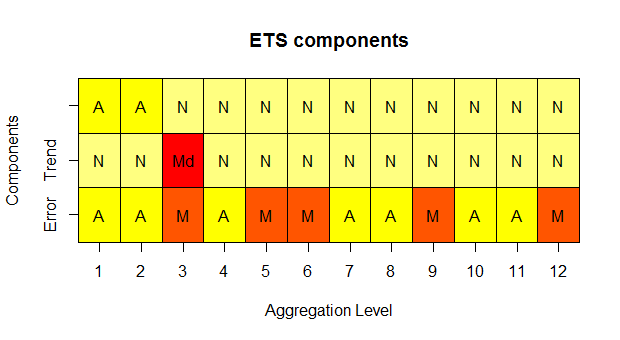The second function provides in- and out-of-sample forecasts. By default only one step ahead in-sample forecasts are given.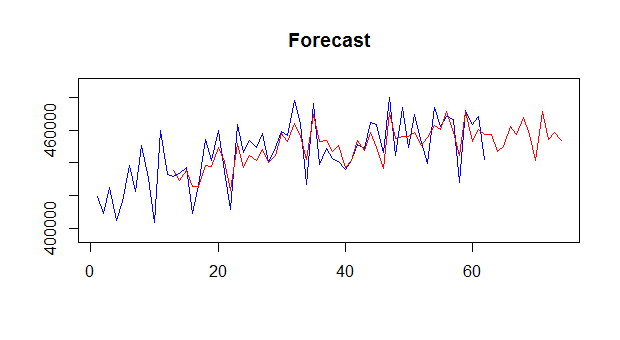This is easy to change, for instance to 12-steps ahead:

`> mapafor(admissions,mapafit,ifh=12,fh=0)`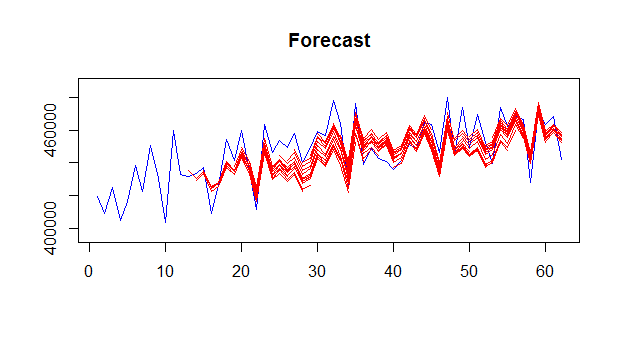I can stop plotting the output by setting outplot=0 in any of the above functions. The functions have are several more options, allowing you to set the maximum temporal aggregation level, the type of MAPA combination, etc. Finally, the function mapa is a wrapper for both mapaest and mapafor, so these can be run with a single call.
Since version 1.5 of the package there are some new interesting features. The first one is to force a particular exponential smoothing model on all aggregation levels. This can be simply done by using the option model:

`> mapa(admissions,model="AAdN",paral=2)`

A nonseasonal damped trend model is fitted to the time series in this case. Since MAPA can no longer change between models and choose a simpler one, it is possible that the preselected model will have too many degrees of freedom for the aggregate version of a given series. In this case no model is fitted.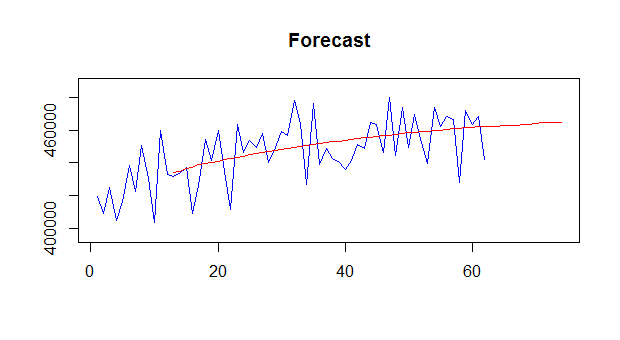Furthermore, if a seasonal model is selected, for any aggregation levels with non=integer seasonality a non-seasonal version of that model will be fitted.
Another new option is the ability to calculate empirical prediction intervals. As these require simulating forecasts for their calculation, they are computationally expensive and not provided by default. To get the 80%, 90%, 95% and 99% prediction intervals you can use:

`> mapa(admissions,conf.lvl=c(0.8,0.9,0.95,0.99),paral=2)`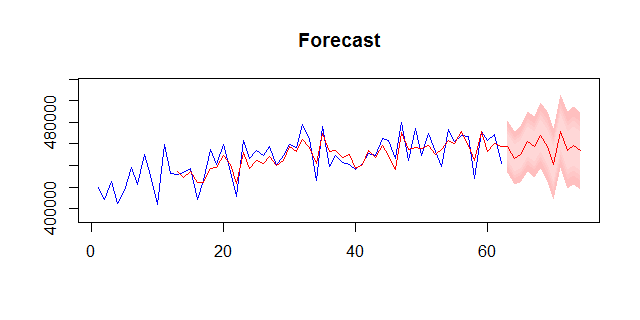For more details about the Multiple Aggregation Prediction Algorithm (MAPA) look at the this post.

Update: The MAPA package now allows modelling high-frequency time series and include regressors (MAPAx).

## 6 thoughts on “Multiple Aggregation Prediction Algorithm (MAPA)”

1.Bhanu Pratap

You had mentioned in your paper that MAPA technique can be used for intermittent demand. I wanted to know, can MAPA R package handle intermittent demand forecasting?

1.Nikos Post author

Hi Bhanu,
Indeed MAPA can handle intermittent demand. However, the MAPA package does not call intermittent methods to produce forecasts. You can use the tsintermittent package for R and the function imapa for this purpose. Alternatively the thief package might be helpful, which can accept any type of forecast and perform multple temporal aggregation.
Good luck!

2.Mike Hawkins

Hi Nikos. Have you thought of a way to combine a weekly and monthly temporal level? For example if you wish to forecast at a monthly level, but you know that there are components of the time series that are stronger at the weekly level. How could you incorporate this into the model? Thanks

1.Nikos Post author

Hi Mike,

Relatively high frequency data is a quite interesting problem!

With MAPA the usual route would be followed, i.e. start from the most highest frequency time series and let the algorithm do the rest. However you would need to switch to “w.mean” or “w.median” for the combination type – these handle better high frequency time series. MAPA would consider all possible aggregations till the annual level (as defined by the argument ppy).

If you would use Temporal Hierarchies (thief package in R), then it really depends how you encode weekly seasonality. Suppose you use a 4-4-5 calendar (weeks in the months of a quarter), resulting in a fixed 52 weeks annual seasonality, then thief would work fully automatically, but it would skip the monthly level, but use a quasi-monthly level! See the interactive diagram here to see exactly which aggregation levels would be considered. If you do not follow a 4-4-5 calendar and you would have a variable number of weeks in a year, then you would need to manually encode the aggregation rules, which would make it substantially more complicated – but also very hard to model, even at the original weekly data with no temporal aggregation.

The forecasts you would get from either MAPA or Temporal Hierarchies would be at a weekly level, but would include all the information from the most aggregate levels. If you would like to constuct the forecasts at the monthly level then you would need to do that by calling function tsaggr (MAPA package) or tsaggregates (thief package).

Hope this helps,
Nikos

3.K

Hi,
I would like to use MAPA package for TS. However when i try to use as suggested above using mapasimple i get result as
“Paper reference:
Kourentzes N., Petropoulos F. and Trapero J.R. (2014)”.
i am not sure what is missing. I have the data(MTM) in TS format uni variate TS(monthly) around 60 observations.
Can you help me in using it?
Thanks

4.K

I have found the error. Should e converted to TS format before applying mapasimple function
Thanks